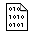## Firefly and PC GAMESS-related discussion club

Learn how to ask questions correctlyDifferences between MCSCF and XMCQDPT2 results

Panwang Zhou
pwzhou@gmail.com

Dear all,

Recently I performed MCSCF(14,12) and related XMCQDPT2 calculations on a molecule where the pipi* and npi* excited states are involved.

1) The active orbitals are 6 pi orbitals, 1 n orbital, and 5 pi* orbitals.

2) The state-averaged calculations are performed with 4 states, that is SA4-MCSCF(14,12)

3) For this molecule, at the optimized s0 and pipi* state, the first and second excited state are pipi* and npi*, respectively. However, at the optimized npi* state, the first excited state is npi* state. A CI is existed between the optimized pipi* and npi* state geometries.

4)The start geometry is closed to that of the npi* state, the HF calculations is first performed and the active space is selected, then a SA4-MCSCF single point energy calculation is performed, when the converged MCSCF result is obtained, the geometry of S0 and npi states are optimized with SA4-MCSCF(14,12) for root 1 and 2. The pipi* state geometry is optimized from the optimized s0 geometry for root 2.

5) With the SA4-MCSCF(14,12), the geometries of s0, pipi*, npi*, and CI between pipi* and npi* states are sucessfully located. The calculated energy profiles is shown in Figure 1 (see attachments).

6) I performed XMC-QDPT2 single point energy calculations on the optimzed geometries of s0, pipi*, npi*, and CI between pipi* and npi* states. The calculated abs. and fluo. spectra are close to the experimetal results (see Figure 2 of the attachment). However, Some of the results are confused for me:

i) At the optimized geometries of s0 and pipi* states, for the MCSCF results, the four states are s0, pipi*, npi* and pipi*. However, for the XMCQDPT2 results, the four states are s0, pipi*, pipi* and npi*. That is to say, the npi* in the MCSCF is the third state and in XMCQDPT2 it is become the fourth state. I confirmed this from the QDPT2 natural orbitals for the four states with ChemCraft. At the optimized geometries of CI and npi* state, the orders of the four states for MCSCF and XMCQDPT2 are the same.

ii) For the QDPT2 natural orbitals, the occ number of the first and second orbitals of active space are 2.00 and 1.99, this is not observed for the MCSCF natural orbitals.

iii) At the CI point, the calculated energy difference with MCSCF is close to 0, however, with the XMCQDPT2, the energy difference is about 18.4 kcal/mol.

My quensiton is: Are the above-metioned XMCQDPT2 results resonable? ĀThanks.

Best Regards!

Panwang ZhouThis message contains the 91 kb attachment [ MMBC_1.zip ]Thu Aug 20 '15 12:21pmThis message read 860 times